## Gaussian Curvature

An intrinsic property of a space independent of the coordinate system used to describe it. The Gaussian curvature of a Regular Surface inat a point p is formally defined as(1)

whereis the Shape Operator and det denotes the Determinant.

Ifis a Regular Patch, then the Gaussian curvature is given by(2)

where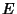,, andare coefficients of the first Fundamental Form and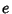,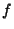, andare coefficients of the second Fundamental Form (Gray 1993, p. 282). The Gaussian curvature can be given entirely in terms of the first Fundamental Form(3)

and the Discriminant(4)

by(5)

where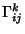are the Connection Coefficients. Equivalently,(6)

where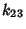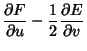(7)(8)

Writing this out,(9)

The Gaussian curvature is also given by(10)

(Gray 1993, p. 285), as well as(11)

where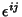is the Levi-Civita Symbol,is the unit Normal Vector andis the unit Tangent Vector. The Gaussian curvature is also given by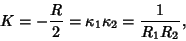(12)

whereis the Curvature Scalar,andthe Principal Curvatures, andandthe Principal Radii of Curvature. For a Monge Patch with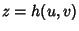,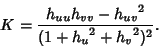(13)

The Gaussian curvatureand Mean Curvaturesatisfy(14)

with equality only at Umbilic Points, since(15)

If p is a point on a Regular Surfaceandandare tangent vectors toat p, then the Gaussian curvature ofat p is related to the Shape Operatorby(16)

Let Z be a nonvanishing Vector Field onwhich is everywhere Perpendicular to, and letand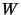be Vector Fields tangent tosuch that, then(17)

(Gray 1993, pp. 291-292).

For a Sphere, the Gaussian curvature is. For Euclidean Space, the Gaussian curvature is. For Gauss-Bolyai-Lobachevsky Space, the Gaussian curvature is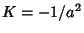. A Flat Surface is a Regular Surface and special class of Minimal Surface on which Gaussian curvature vanishes everywhere.

A point p on a Regular Surfaceis classified based on the sign ofas given in the following table (Gray 1993, p. 280), whereis the Shape Operator.

 Sign PointElliptic PointHyperbolic Point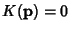but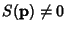Parabolic PointandPlanar Point

A surface on which the Gaussian curvatureis everywhere Positive is called Synclastic, while a surface on whichis everywhere Negative is called Anticlastic. Surfaces with constant Gaussian curvature include the Cone, Cylinder, Kuen Surface, Plane, Pseudosphere, and Sphere. Of these, the Cone and Cylinder are the only Flat Surfaces of Revolution.

See also Anticlastic, Brioschi Formula, Developable Surface, Elliptic Point, Flat Surface, Hyperbolic Point, Integral Curvature, Mean Curvature, Metric Tensor, Minimal Surface, Parabolic Point, Planar Point, Synclastic, Umbilic Point

References

Geometry Center. Gaussian Curvature.'' http://www.geom.umn.edu/zoo/diffgeom/surfspace/concepts/curvatures/gauss-curv.html.

Gray, A. The Gaussian and Mean Curvatures'' and Surfaces of Constant Gaussian Curvature.'' §14.5 and Ch. 19 in Modern Differential Geometry of Curves and Surfaces. Boca Raton, FL: CRC Press, pp. 279-285 and 375-387, 1993.